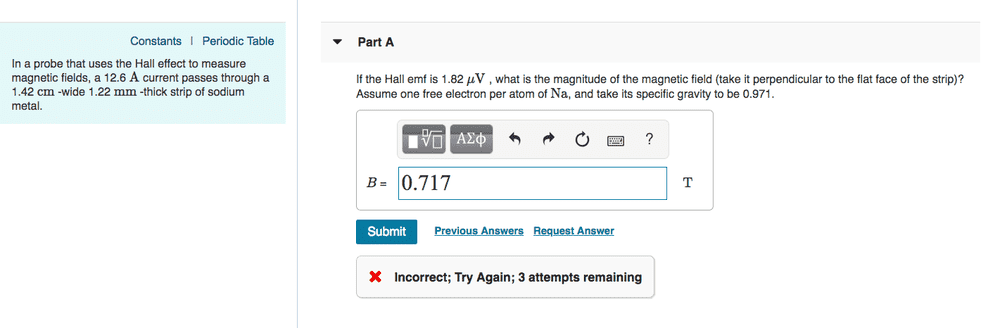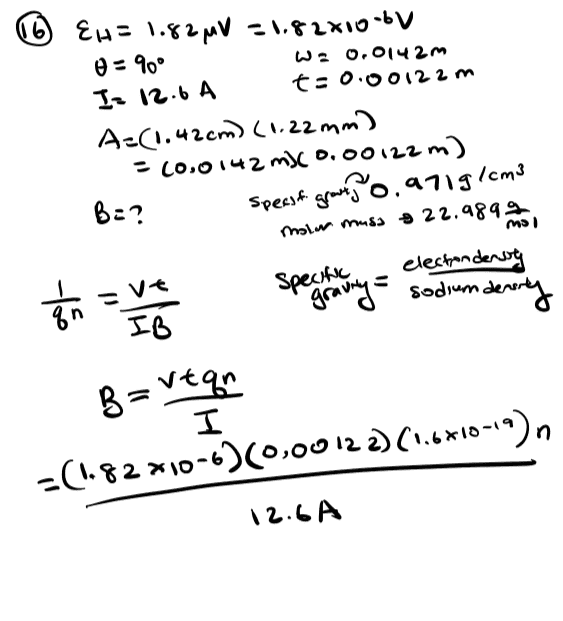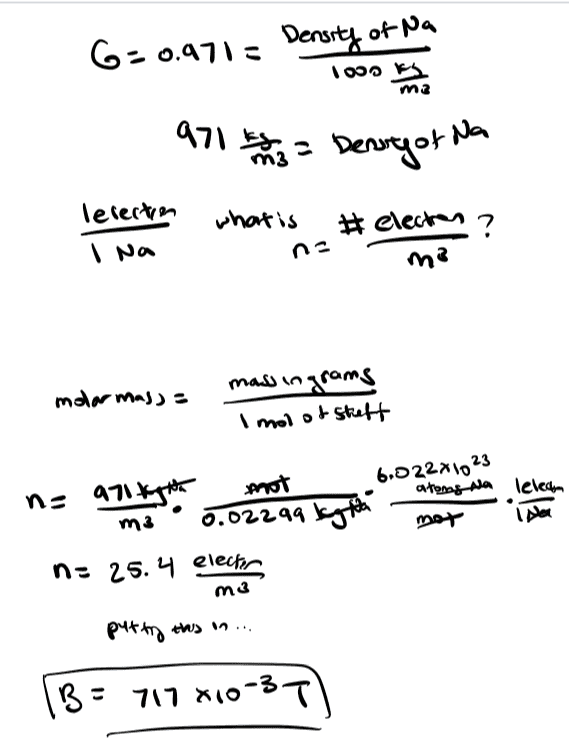# Find the magnetic field given I, t, Hall EMF, and G_s

• lumealume

#### lumealume

Hi! I had this problem for homework (Mastering Physics), and I'm not sure what I'm doing incorrectly. Is there something fundamental that I'm misunderstanding? Each time I do this, I get 0.717136.. T as my solution

1. Homework StatementI = 12.6 A
w = 0.0142 m
t = 0.00122 m
E_H = 1.82 * 10^(-6) V
G_s = 0.971
B = ?

1/(qn) = Vt/(IB)

## The Attempt at a Solution

1. I have the formula for B from 1/(qn) = Vt/(IB) => B = Vtqn/I
2. Plug V, t, q (electron charge), and I into get B = (1.82 * 10^(-6) V)(0.00122 m)(1.6 * 10^(-19)) * n / 12.6 A
3. Now it's time to solve for n: G_s = 0.971 = (Density of Na)/(Density of water) = (Density of Na)/(1000 kg/m^3)
4. Density of Na = 971 kg/m^3
5. I want n = electron density in units of electrons / m^3.
6. There is 1 electron per 1 atom of Na so...
7. n = Density of Na * mols of Na per mass in kg * Na atoms per mol * electron per Na atom will cancel everything out nicely to get me electrons / m^3
8. n = (971 kg/m^3) * (mol/(0.02299kg)) * (6.022*10^23 atoms of Na / mol) * (1 electron / 1 atom Na)
9. Putting it all together: B = (1.82 * 10^(-6) V)(0.00122 m)(1.6 * 10^(-19)) * (971 kg/m^3) * (mol/(0.02299kg)) * (6.022*10^23 atoms of Na / mol) * (1 electron / 1 atom Na) / 12.6 A
10. B = 0.717 TEDIT: (editing the post currently because I just realized that I shouldn't just post images. I'm new here!)
EDIT2: Finished editing post with thought process

Last edited: